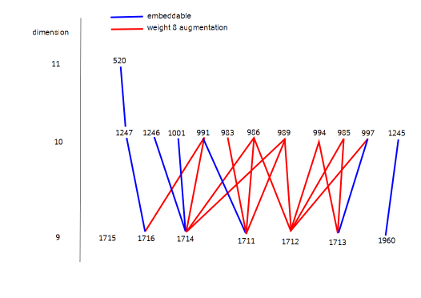## DATABASE : Triply Even Codes of Length 48

Last update 2017/03/21
Update 2011/6/29
A triply even code is a binary code in which the weight of every codeword is divisible by 8.
In this page, a triply even code is a triply even code which contains the all-ones vector.
We thank Dr. Michael Kiermaier whose comment prompted us to clarify this additional assumption we have made in the classification.

### An Enumeration of triply even codes of length 48 up to equivalence

Dimension   Number of codes
11
23
310
435
5136
6458
71162
81910
91960
101247
11520
12159
1339
146
151
There precisely exist 3 triply even codes which are not subcodes of doublings.
Dimension           ID                remark
91 The triangular code
81
73

### Paper and Magma Script

The paper of this project "On triply even binary codes" is available at
arxiv [1012.4134].

The magma script to obtain this result is
classification.tex
This file is excutable on Magma as follows:
> magma classification.tex

It is available on Latex as well with the file:
triply-even.tex.
One can obtain the script with a reader friendly format and comments by executing command
> latex triply-even.tex

### Generator Matrices

The following files give a complete list of triply even codes of length 48 up to equivalence.

The generator matrices are given by the heximal form in
File of Generator Matrix [Heximal form | Matrix form | Magma form].

Each code is described by the form
<Dimension, Code Id, [ Generators ]>

For example, the 132nd code of dimension 5 is descrebed by the heximal form and the matrix form respectively as follows:

<5, 132, [ 0x9669, 0xFFFFFFFFAAAA, 0xFFFFFFFFCCCC, 0xFFFFFFFFF0F0, 0xFF00 ]>,

<5, 132, [48, 5, 8]




>,


### Profiles of codes

#### Automorphism Groups

The following files give the composition factors of automorphism groups of codes.

Dimension = 1, 2, 3, 4, 5, 6, 7, 8, 9, 10, 11, 12, 13, 14, 15

#### Weight Enumerators

The following files give the weight enumerators of codes.
Each weight enumerator is described by a list of pair of the degree and coefficient of each monomial. For example, <3, 4, [ <0, 1>, <24, 6>, <48, 1> ]> indicates $1+6x^{24}+x^{48}$.

Dimension = 1, 2, 3, 4, 5, 6, 7, 8, 9, 10, 11, 12, 13, 14, 15

### Characterizations of codes

#### Doubling Form

The list of codes constructed by doubling is given by File of the list of doublings.

The format is as follows
$\langle k, [ \langle\mathrm{id}_1,\mathrm{id}'_1\rangle, \langle\mathrm{id}_2,\mathrm{id}'_2\rangle,\ldots, \langle\mathrm{id}_n,\mathrm{id}'_n\rangle]\rangle$
$\mathrm{id}_i$ indecates the code $C_{\mathrm{id}_i}$ of dimension $k$ which is equivalent to a code of the form $\{(c \mid c) \mid c \in C\}$
and $\mathrm{id}'_i$ indecates the code $C'_{\mathrm{id}'_i}$ of dimension $k+1$ which is equivalent to $C_{\mathrm{id}_i} + \langle (0,\ldots,0,1,\ldots,1)\rangle$.

#### Extended Doubling Form

The list of codes constructed by extended doubling is given by File of the list of extended doublings.

The format is as follows
$\langle k,\mathrm{id}\rangle, [ \langle k_1,\mathrm{id}_1\rangle, \langle k_2,\mathrm{id}_2\rangle,\ldots, \langle k_m,\mathrm{id}_m\rangle]\rangle$
where $\langle k_i,\mathrm{id}_i\rangle$ indecates the code $C_{\mathrm{id}_i}$ of dimension $k_i$ which is equivalent to a code of the form $\{(c \mid c) \mid c \in C\}$
and $C_{\mathrm{id}}$ is an exteded doubling code constructed from each code $C_{\mathrm{id}_i}$,
which is equivalent to $C_{\mathrm{id}_i} + \langle (0 \mid D )\rangle$ where $C_{\mathrm{id}_i} =\{(c\mid c) \mid c \in C\}$ and $D=\mathrm{Rad}\; C$.

#### Non-Embeddable to Doubling

File of the list of non-embeddable codes gives the list of codes non-embeddable in doublings.

#### Decomposability

The list of decomposable codes is given in File of decomposable codes.

Each component is given in File of components of decomposable codes.

### Dual Weight is >= 4

• The list of all codes : dw4.txt
• The list of embeddable into a doubling : dw4embeddable.txt
• The list of realizable : realizable.txt (calculated by Munemasa).
• The list of non embeddable : dw4nonembeddable.txt
• The relations Embeddable by the weight 8 augmentation is given in w8ext.txt.
An entry $\langle k,\mathrm{id}_1, \mathrm{id}_2\rangle$ indecats that the code $C_{\mathrm{id}_2}$ of dimension $k+1$
can be obtained from the code $C_{\mathrm{id}_1}$ of dimension $k$ by attaching some weight 8 vector.
• Tracable codes via the relation above (embeddalbe by weight 8 augmentation) is given in w8tracable.txt.
• Remaining from the trace above is given in undetermined.txt.
• The embeddable relation among the remaining codes in undetermined.pdf.
•### Maximal triply even codes

The following codes are the maximal triply even codes.
• <15,1> : Three copies of $\mathrm{RM}(1,4)$
• <14,1> : Direct sum of $\mathrm{RM}(1,4)$ and doubling of $d_{16}^{+}$.
• <13,1> : Doubling of $g_{24}$ of order of Aut is 1002795171840.
• <13,2> : Doubling of $(d_{10}e_7^2)^{+}$ of order of Aut is 443925135360.
• <13,3> : Doubling of $d_{24}^{+}$ of order of Aut is 12054469961318400.
• <13,4> : Doubling of $d_{12}^{2+}$ of order of Aut is 4348654387200.
• <13,5> : Doubling of $d_4^{6+}$ of order of Aut is 36238786560.
• <13,6> : Doubling of $d_6^{4+}$ of order of Aut is 32614907904.
• <13,7> : Doubling of $d_8^{3+}$ of order of Aut is 173946175488.
• <9,1> : The code induced from the triangular graph.
• ### Well known triply even code

• <7,144> : The Miyamoto's moonshine code, which contains the MacDonald [48, 6, 24] code.